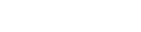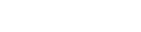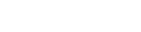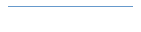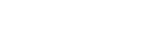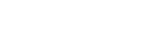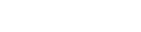This page contains answers to common questions handled by our support staff, along with some tips and tricks that we have found useful and presented here as questions.How do I ... ?Where can I find ... ?Why doesn't ... ?Who is ... ?What is ... ?When is ... ?#### How do I ... ?

This is the answer to the question. This is the answer to the question. This is the answer to the question. This is the answer to the question. This is the answer to the question. This is the answer to the question. This is the answer to the question. This is the answer to the question.#### Where can I find ... ?

This is the answer to the question. This is the answer to the question. This is the answer to the question. This is the answer to the question. This is the answer to the question. This is the answer to the question. This is the answer to the question. This is the answer to the question.#### Why doesn't ... ?

This is the answer to the question. This is the answer to the question. This is the answer to the question. This is the answer to the question. This is the answer to the question. This is the answer to the question. This is the answer to the question. This is the answer to the question.#### Who is ... ?

This is the answer to the question. This is the answer to the question. This is the answer to the question. This is the answer to the question. This is the answer to the question. This is the answer to the question. This is the answer to the question. This is the answer to the question.#### What is ... ?

This is the answer to the question. This is the answer to the question. This is the answer to the question. This is the answer to the question. This is the answer to the question. This is the answer to the question. This is the answer to the question. This is the answer to the question.

This is the answer to the question. This is the answer to the question. This is the answer to the question. This is the answer to the question. This is the answer to the question.#### When is ... ?

This is the answer to the question. This is the answer to the question. This is the answer to the question. This is the answer to the question. This is the answer to the question. This is the answer to the question. This is the answer to the question. This is the answer to the question.

This is the answer to the question. This is the answer to the question. This is the answer to the question. This is the answer to the question. This is the answer to the question.Home | M & M Carpets, Inc. | Products | F.A.Q. | Service Request | Comments | Catalogs / Manuals | Support Forum Questions or problems regarding this web site should be directed to [CompanyEmail]. Copyright © 2002 [CompanyName]. All rights reserved. Last modified: 07/02/01.